# Glossary

(diff) ← Older revision | Latest revision (diff) | Newer revision → (diff)

These are key concepts and terms used in Analytica.

## Influence Diagrams

Arrow tool: A tool available from the Toolbar, looking like this. It lets you draw influence arrows between nodes.

Influence diagram: A graphical representation of a model, consisting of nodes (variables) and arrows (relationships between variables).

Node: A box (rectangular, oval, or any other shape) that represents a variable in an influence diagram. Different node shapes are used to represent different types of variables.

Arrow: An arrow from one variable node to another in a Diagram depicts an Influence -- i.e. that the origin node affects (influences) the destination node. If the nodes depict variables, the origin variable is an Input to the destination variable, and the destination variable is an Output of the origin variable.

Influence arrow: See Arrow, influence arrow.

Shows that a node has one or more inputs from outside its module. This arrowhead is located on the left side of a node. Press the arrowhead for a popup menu of input variables.
Shows that a node has one or more outputs outside its module. This arrowhead is located on the right side of the node. Press the arrowhead for a popup menu of the output variables.
User Input
An input of a variable X is a variable that has an arrow drawn to X, or appears in the definition of X. See also User Output.
User Output
An output of a variable X is a variable that has an arrow drawn from X, or whose definition refers to X. See also User Input.

## Attributes of an Object

Attribute: A property of an object, such as its title, description, definition, value, or inputs.

Class: An Attribute of an Object defining its type. Each Class of Object has its own node shape in a Diagram. Object Classes include Variable (with subclasses decision, chance, objective, index, constant, constraint, and general variables), Module (with subclasses Model, Module, Filed Module, Form Module), Function, and Attribute. You can modify the Class of an Object (within its Subclass) using the Class menu at the top left of an Object View, or in the Attribute Pane when you select the Class attribute.

Definition: An Attribute of a Variable or Function that contains an expression used to calculate the Value of the Variable or Function. A expression can be a single number or text value, a formula using operators or function calls, a list of values, a table, or a probability distribution.

Description: An Attribute of a Variable or other object with text explaining what the object represents. Descriptions, like other text attributes, may be up to 32,000 characters long.

Domain
The possible outcomes for a variable defined as a probability table.
Identifier
A short name for an object. A variable’s identifier is used to refer to the variable in mathematical expressions in definitions of other variables. An identifier must start with a letter, have no more than 20 characters, and contain only letters, numbers, and the underscore character (_) (used instead of a space). Compare to Title.
Inputs
Variables that an Analytica object uses to calculate the Value of the Variable or Function.
Outputs
Variables that rely on the Analytica object in order to calculate their Values.
Title
The full name of an Analytica object, briefly describing what the object represents. The title of a variable, function, or module is displayed in its node, in window titles, and in object lists. It is limited to 255 characters in length. The title can contain any characters, including spaces and punctuation. Compare to Identifier.
Units
The increments of measurement for a variable. Units are used to annotate tables and graphs, but are not used in evaluating a variable.
Value
A variable’s value attribute is its mid value, computed by assuming that all uncertain inputs are fixed at their median values. It can be a scalar (single) number, a table of numbers, or a probability distribution.

## Types of Analysis

Importance analysis
Shows the effect the uncertainty of one or more input variables has on the uncertainty of an out- put variable. Importance is defined as the rank-order correlation between the sample of output values and the sample for each uncertain input. This is a robust measure of the uncertain contri- bution because it is insensitive to extreme values and skewed distributions.
Sensitivity analysis
A group of methods to identify and compare the effects of various input variables to a model on a selected output. Example methods of sensitivity analysis are importance analysis and parametric analysis. See also Parametric analysis.
Parametric analysis
A type of sensitivity analysis in which you specify a set of alternative values for one or more inputs, and examine the effect on selected model output variables. See also Sensitivity analysis.
Uncertainty analysis

## Classes of Object Variables

Chance variable: A Class of Variable whose value is uncertain. It is depicted as an oval node: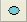. Usually a Chance Variable contains a probability distribution in its Definition. A decision maker cannot directly change the value of a Chance Variable, unlike a Decision Variable.

Decision variable: A variable that the decision maker can control directly. A decision variable appears in a Diagram as a rectangular green node: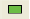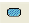General variable
Any type of variable; useful when the variable type is unknown. The general variable typically rep- resents a deterministic or functional dependency.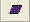Index variable
A class of variable, defined as a list, list of labels, or sequence, that is used to identify the dimensions of a table, for example, in an edit table. An index variable is represented by a parallelogram node. See also Edit table.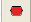Objective variable
A variable that evaluates the overall value or desirability of possible outcomes. The objective can be measured as cost, value, or utility. A purpose of most models is to find the decision or deci- sions that optimize the objective — for example, minimizing cost or maximizing expected utility. Most decision models contain a single objective node, although the objective can be composed of several sub-objectives. An objective variable is represented by a hexagonal node.
Probabilistic variable
A variable that is uncertain and is defined with a probability distribution.
Variable
An object that has a value, which can be a text string, a number, or a table. Classes of variable include decision, chance, and objective.
Model
A module, or a hierarchy of modules; the main, or root, module at the top of the module hierarchy. Between sessions, a model is stored in an Analytica document file.Module
A collection of related objects, including variables, functions, and other modules, organized as a separate influence diagram. A module is represented by a rounded rectangular node with a thick outline.

Node: A box (rectangular, oval, or any other shape) that represents a variable in an influence diagram. Different node shapes are used to represent different types of variables.

Object: A Model consists of a set of Objects of various Classes, including Variables, Modules, Functions, Text Objects, and more. Each Object appears as a node in in its parent Diagram.

## Statistics

Deterministic value
Same as Mid value: The value of a variable using the median of any probability distribution it contains, and Deterministic (Mid) value of any Input Variables.
Cumulative probability distribution or Cumulative Distribution Function (CDF)
A graphical view of a probability distribution. It shows for each value of X, the probability that x is less than or equal to X. The cumulative probability distribution is a display option in the Uncertainty View popup menu in a Result window.
Exceedance probability
A graphical view of a probability distribution, which shows for each value of X, the probability that x is greater than or equal to X. It is a dual to the Cumulative distribution function (CDF). Starting in Analytica 5.4, the exceedance probability is a display option in the Uncertainty View popup menu in a Result window.
Mean
The average or expected value.
Median
The middle number or value when the data values are ranked in order of size, i.e., the middle data point.
Mid, Mid value
A calculation of the variable’s value assuming all uncertain inputs are fixed at their median values.
Probability bands
The bands that capture a certain portion of the total probability for a variable. For example, the 5% and 95% probability bands contain 90% of the total probability, while the 50% probability band corresponds to the median value. By default, the 5%, 25%, 50%, 75%, and 95% probability bands are shown. These bands are also referred to as confidence intervals or fractiles. Probability bands are a display option in the Uncertainty View popup menu on a Result window.
Probability density function (PDF)
A graphical representation of a probability distribution that plots the probability density against the value of the variable. The probability density at each value of X is the relative probability that X is at or near that value. The probability density function can be displayed for continuous, but not discrete, variables. It is a display option in the Uncertainty View popup menu on a Result window.
Standard deviation
Reflects the amount of spread or dispersion in an uncertainty distribution. It is the square root of the variance.

## Distributions

Normal distribution
The bell-shaped curve, or Gaussian distribution.
Probability distribution
Describes the relative likelihood of a variable having different possible values.
Uniform distribution
A distribution representing an equal chance of occurrence for any value between the lower and upper values.

## Functions

Array: A collection of values that can be viewed as one or more tables. An array has one or more dimensions; each dimension is identified by an index.

Deterministic table
A function that gives the value of a variable conditional on the values of its input variables, where the inputs are all discrete variables.
Edit table
A definition that is an array (table) is also called an edit table because it can be edited.
Expression
A formula that can contain any combination of numbers, variables, functions, distributions, and operators, such as 0.5, a-b, or Min(x), combined according to the Analytica language syntax. Expression type Expression types include expression, list (of expressions or numbers), list of labels (text strings), table, probability table, and distribution. You select an expression type using the Expression popup menu, which appears above the Definition field. Note that any definition, regardless of expression type, can be viewed as an expression.
List
A type of expression, consisting of an ordered set of numbers or expressions, available in the Expression popup menu. A list is often used to define index and decision variables.
List of labels
A type of expression, consisting of an ordered set of text labels, available in the Expression popup menu. A list of labels is often used to define index and decision variables.
Probability table
A table for defining a chance variable with a set of outcomes and a discrete probability distribution (numerical probability for each outcome). If the variable depends on (that is, is conditioned by) other discrete variables, each of these conditioning variables gives an additional dimension to the table, so you can specify the probability distribution conditional on the value of each conditioning variable.
Self
A keyword referring to the index of a table that is indexed by itself. Self refers to the alternative values of the variable defined by the table.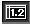Table
A two-dimensional view of an array-valued definition or result. The array can have more than two dimensions, but only two can be seen at one time. A table is a type of expression available in the Expression popup menu. A definition that is a table is also called an edit table. In the Result window, click the table button to select the table view of an array-valued result. See also Graph.

## View Windows

Diagram window:
Attribute panel: An auxiliary window pane that you can open below an influence diagram. It lets you view any attribute of a node, variable, function, or module, that you select in the Diagram.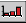Graph: Format for displaying a multidimensional result. To view a result as a graph, click the Graph but- ton. See also Table.
Object Finder: A dialog box used to browse and edit the functions and variables available in a model.
Object window: List of the attributes for an object (variable, function, or module), including its class, identifier, title, and description.
Uncertainty View options: An uncertain result can be displayed as a mid value, mean, statistics, probability bands, probability density, cumulative probability, or sample. Select the option to display with the popup menu in the top left corner of the Result window or in the Result menu.

## Toolbar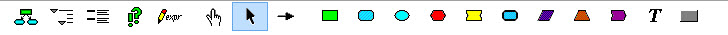Browse tool: A tool in the toolbar looking like this:In Browse mode, you can explore and examine the structure and assumptions of a model, change input values, and view results, but you can't edit the model definitions or modify diagrams.

Edit tool: A tool for creating or changing a model. It appears in the toolbar as: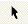After clicking the Edit tool, you can move, resize, and edit nodes. You can also see the arrow tool and node palette that lets you add new nodes.